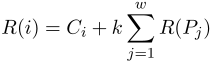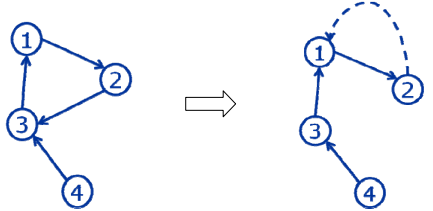## Day 2, Problem 1 - Olympic Logistics

The Beijing 2008 Olympics is about to open, and the entire nation is preparing for this grand event. To maximize efficiency when setting up and hosting a successful Olympics, the planning of logistics is essential.

The logistics system consists of N total stations numbered from 1 to N. Each station i has exactly one successor station Si, but can have many predecessor stations. Station i needs to continuously deliver materials to successor station Si. Obviously, a station's successor station cannot be itself. Station 1 is known as the control station, and any other station can deliver materials to it. Note that the control station also has its own successor station to permit the flow of materials when necessary. In the design of this logistics system, high reliability and low costs are the main objectives. So for station i, we can define its "reliability" R(i) as follows:

Let station i have w predecessor stations P1, P2, …, Pw, meaning that i is the sole successor of each of these station. Then, station i's reliability R(i) is given by the following formula:$\displaystyle R(i) = C_i + k\sum_{j=1}^{w} R(P_j)$

where Ci and k are positive real constants, and k < 1.

The reliability of the entire logistics system is directly based upon the reliability of the control station. Our goal is to maximize the control station's reliability value R(1) by modifying the successor stations of some other stations. However, our budget is also limited – we may only modify at most m successor stations. Furthermore, the successor of the control station cannot be modified. Thus, the overall problem we face is to maximize the control station's reliability R(1) by changing the successors of no more than m stations.

### Input Format

The first line of input contains two integers N and m, followed by one real number k. N represents the number of stations, m represents the maximum number of successor stations that may be changed, and k is the constant used in the definition of the reliability formula.
The second line contains N integers S1, S2, …, SN, representing the successor stations of each station.
The third line contains N positive real numbers C1, C2, …, CN, representing the constant values used in the reliability formula.

### Output Format

The output should contain one real number, the maximum possible value of R(1) rounded and displayed to 2 decimal places.

### Sample Input

4 1 0.5
2 3 1 3
10.0 10.0 10.0 10.0


### Sample Output

30.00


### Explanation

The original logistics system is depicted in the left figure below. There are 4 stations with reliability values 22.8571, 21.4286, 25.7143, and 10, respectively.The best solution is to make the successor of station 2 to station 1, as depicted in the right figure above. The new reliability values of the stations are 30, 25, 15, and 10, respectively.

### Constraints

Test CaseNm
1≤ 6≤ 6
2≤ 12≤ 12
3≤ 600
4≤ 601
5≤ 60N − 2
6 ~ 10≤ 60≤ 60

For all of the test cases, mN ≤ 60,  Ci ≤ 106, and 0.3 ≤ k < 1. Please use double precision real numbers; there is no need to worry about their precision.

Point Value: 25 (partial)
Time Limit: 1.00s
Memory Limit: 128M